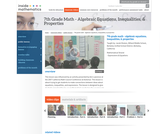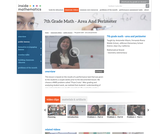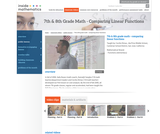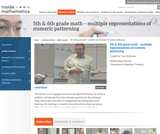# Search Results (40)

View
Selected filters:
• Inside MathematicsConditions of Use:
Read the Fine Print
Rating

This lesson is about trying to get students to make connections between ideas about equations, inequalities, and expressions. The lesson is designed to give students opportunities to use mathematical vocabulary for a purpose to describe, discuss, and work with these symbol strings.The idea is for students to start gathering global information by looking at the whole number string rather than thinking only about individual procedures or steps. Hopefully students will begin to see the symbol strings as mathematical objects with their own unique set of attributes. (7th Grade Math)

Subject:
Algebra
Numbers and Operations
Material Type:
Activity/Lab
Lecture
Lesson Plan
Teaching/Learning Strategy
Provider:
Noyce Foundation
Provider Set:
Inside Mathematics
Author:
Disston, Jacob
Date Added:
11/30/2011Conditions of Use:
Read the Fine Print
Rating

This lesson is based on the results of a performance task in which we realized that students' understanding of area and perimeter was mostly procedural. Therefore the purpose of this re-engagement lesson was to address student misconceptions and deepen student understanding of area and perimeter. The standards addressed in this lesson involve finding perimeter and area of various shapes, finding the perimeter when given a fixed area, and using a formula in a practical context. Challenges for our students included decoding the language in the problem and proving their thinking. (7th Grade Math)

Subject:
Mathematics
Material Type:
Activity/Lab
Lecture
Lesson Plan
Teaching/Learning Strategy
Provider:
Noyce Foundation
Provider Set:
Inside Mathematics
Author:
Villarin, Antoinette
Date Added:
11/30/2011Conditions of Use:
Read the Fine Print
Rating

The foundation of this lesson is constructing, communicating, and evaluating student-generated tables while making comparisons between three different financial plans. Students are given three different DVD rental plans and asked to analyze each one to see if they could determine when the 3 different DVD plans cost the same amount of money, if ever. (7th/8th Grade Math)

Subject:
Algebra
Material Type:
Activity/Lab
Lecture
Lesson Plan
Teaching/Learning Strategy
Provider:
Noyce Foundation
Provider Set:
Inside Mathematics
Author:
Dimas, Cecilio
Date Added:
11/30/2011Conditions of Use:
Read the Fine Print
Rating

This lesson is a re-engagement lesson designed for learners to revisit a problem-solving task they have already experienced. Students will activate prior knowledge of graphical representations through the 'what's my rule' number talk; compare and contrast two different learners' interpretations of the growing pattern; use multiple representations to demonstrate how one of these learners would represent the numeric pattern; make connections between the different representations to more critically compare the two interpretations. (5th/6th Grade Math)

Subject:
Numbers and Operations
Material Type:
Activity/Lab
Lecture
Lesson Plan
Teaching/Learning Strategy
Provider:
Noyce Foundation
Provider Set:
Inside Mathematics
Author:
Dickinson, Fran
Date Added:
11/30/2011Rating

When you add two numbers you get a certain answer. Using the same two numbers, subtract the smaller from the larger number. If the two answers are the same, we will call that a perfect pair. This is a problem of the month from Inside Mathematics.

Subject:
Algebra
Material Type:
Activity/Lab
Provider:
Noyce Foundation
Provider Set:
Inside Mathematics
Date Added:
12/09/2013Conditions of Use:
Read the Fine Print
Rating

These geometry and measurement problems of the month are designed to be used schoolwide to promote a problem-solving theme at your school. Each problem is divided into five levels, Level A through Level E, to allow access and scaffolding for the students into different aspects of the problem and to stretch students to go deeper into mathematical complexity.

Subject:
Geometry
Material Type:
Activity/Lab
Provider:
Noyce Foundation
Provider Set:
Inside Mathematics
Date Added:
11/30/2011Conditions of Use:
Read the Fine Print
Rating

These number, geometry, and measurement problems of the month are designed to be used schoolwide to promote a problem-solving theme at your school. Each problem is divided into five levels, Level A through Level E, to allow access and scaffolding for the students into different aspects of the problem and to stretch students to go deeper into mathematical complexity.

Subject:
Geometry
Material Type:
Activity/Lab
Provider:
Noyce Foundation
Provider Set:
Inside Mathematics
Date Added:
11/30/2011Conditions of Use:
Read the Fine Print
Rating

These geometry problems of the month are designed to be used schoolwide to promote a problem-solving theme at your school. Each problem is divided into five levels, Level A through Level E, to allow access and scaffolding for the students into different aspects of the problem and to stretch students to go deeper into mathematical complexity.

Subject:
Geometry
Material Type:
Activity/Lab
Provider:
Noyce Foundation
Provider Set:
Inside Mathematics
Date Added:
11/30/2011Conditions of Use:
Read the Fine Print
Rating

These geometry problems of the month are designed to be used schoolwide to promote a problem-solving theme at your school. Each problem is divided into five levels, Level A through Level E, to allow access and scaffolding for the students into different aspects of the problem and to stretch students to go deeper into mathematical complexity.

Subject:
Geometry
Material Type:
Activity/Lab
Provider:
Noyce Foundation
Provider Set:
Inside Mathematics
Date Added:
11/30/2011Conditions of Use:
Read the Fine Print
Rating

These algebra problems of the month are designed to be used schoolwide to promote a problem-solving theme at your school. Each problem is divided into five levels, Level A through Level E, to allow access and scaffolding for the students into different aspects of the problem and to stretch students to go deeper into mathematical complexity. Algebra problems cover number functions and algebraic reasoning.

Subject:
Algebra
Material Type:
Activity/Lab
Provider:
Noyce Foundation
Provider Set:
Inside Mathematics
Date Added:
11/30/2011Conditions of Use:
Read the Fine Print
Rating

These number and algebra problems of the month are designed to be used schoolwide to promote a problem-solving theme at your school. Each problem is divided into five levels, Level A through Level E, to allow access and scaffolding for the students into different aspects of the problem and to stretch students to go deeper into mathematical complexity.

Subject:
Algebra
Material Type:
Activity/Lab
Provider:
Noyce Foundation
Provider Set:
Inside Mathematics
Date Added:
11/30/2011Conditions of Use:
Read the Fine Print
Rating

These data analysis problems of the month are designed to be used schoolwide to promote a problem-solving theme at your school. Each problem is divided into five levels, Level A through Level E, to allow access and scaffolding for the students into different aspects of the problem and to stretch students to go deeper into mathematical complexity. The problems cover statistics, probability, discrete math, and counting principles.

Subject:
Statistics and Probability
Material Type:
Activity/Lab
Provider:
Noyce Foundation
Provider Set:
Inside Mathematics
Date Added:
11/30/2011Conditions of Use:
Read the Fine Print
Rating

These measurement problems of the month are designed to be used schoolwide to promote a problem-solving theme at your school. Each problem is divided into five levels, Level A through Level E, to allow access and scaffolding for the students into different aspects of the problem and to stretch students to go deeper into mathematical complexity. Measurement problems cover number rate, area, and perimeter.

Subject:
Mathematics
Material Type:
Activity/Lab
Provider:
Noyce Foundation
Provider Set:
Inside Mathematics
Date Added:
11/30/2011Conditions of Use:
Read the Fine Print
Rating

These data analysis problems of the month are designed to be used schoolwide to promote a problem-solving theme at your school. Each problem is divided into five levels, Level A through Level E, to allow access and scaffolding for the students into different aspects of the problem and to stretch students to go deeper into mathematical complexity. The problems cover statistics, probability, discrete math, and counting principles.

Subject:
Numbers and Operations
Material Type:
Activity/Lab
Provider:
Noyce Foundation
Provider Set:
Inside Mathematics
Date Added:
11/30/2011Conditions of Use:
Read the Fine Print
Rating

These data analysis problems of the month are designed to be used schoolwide to promote a problem-solving theme at your school. Each problem is divided into five levels, Level A through Level E, to allow access and scaffolding for the students into different aspects of the problem and to stretch students to go deeper into mathematical complexity. The problems cover statistics, probability, discrete math, and counting principles.

Subject:
Statistics and Probability
Material Type:
Activity/Lab
Provider:
Noyce Foundation
Provider Set:
Inside Mathematics
Date Added:
11/30/2011Conditions of Use:
Read the Fine Print
Rating

These number, algebra, and data analysis problems of the month are designed to be used schoolwide to promote a problem-solving theme at your school. Each problem is divided into five levels, Level A through Level E, to allow access and scaffolding for the students into different aspects of the problem and to stretch students to go deeper into mathematical complexity.

Subject:
Algebra
Material Type:
Activity/Lab
Provider:
Noyce Foundation
Provider Set:
Inside Mathematics
Date Added:
11/30/2011Conditions of Use:
Read the Fine Print
Rating

These algebra problems of the month are designed to be used schoolwide to promote a problem-solving theme at your school. Each problem is divided into five levels, Level A through Level E, to allow access and scaffolding for the students into different aspects of the problem and to stretch students to go deeper into mathematical complexity. Algebra problems cover number functions and algebraic reasoning.

Subject:
Algebra
Material Type:
Activity/Lab
Provider:
Noyce Foundation
Provider Set:
Inside Mathematics
Date Added:
11/30/2011Conditions of Use:
Read the Fine Print
Rating

These measurement problems of the month are designed to be used schoolwide to promote a problem-solving theme at your school. Each problem is divided into five levels, Level A through Level E, to allow access and scaffolding for the students into different aspects of the problem and to stretch students to go deeper into mathematical complexity. Measurement problems cover number rate, area, and perimeter.

Subject:
Mathematics
Material Type:
Activity/Lab
Provider:
Noyce Foundation
Provider Set:
Inside Mathematics
Date Added:
11/30/2011Conditions of Use:
Read the Fine Print
Rating

These measurement problems of the month are designed to be used schoolwide to promote a problem-solving theme at your school. Each problem is divided into five levels, Level A through Level E, to allow access and scaffolding for the students into different aspects of the problem and to stretch students to go deeper into mathematical complexity. Measurement problems cover number rate, area, and perimeter.

Subject:
Mathematics
Material Type:
Activity/Lab
Provider:
Noyce Foundation
Provider Set:
Inside Mathematics
Date Added:
11/30/2011Conditions of Use:
Read the Fine Print
Rating

These algebra and measurement problems of the month are designed to be used schoolwide to promote a problem-solving theme at your school. Each problem is divided into five levels, Level A through Level E, to allow access and scaffolding for the students into different aspects of the problem and to stretch students to go deeper into mathematical complexity.

Subject:
Algebra
Material Type:
Activity/Lab
Provider:
Noyce Foundation
Provider Set:
Inside Mathematics
Date Added:
11/30/2011